##Line Involution on a line and circle bundle generated

There is a basic figure by which we define involutive homographies on lines. This is done by intersecting a line (e) with the six sides of a complete quadrilateral. The three pairs of points (X1,X2), (Y1,Y2) and (Z1,Z2) intercepted on (e) by corresponding pairs of opposite sides of the quadrangle are in involution. This is discussed in InvolutionBasic.html .
Here we consider the three circles with diameters the segments (X1X2), (Y1Y2) and (Z1Z2). They belong to the same circle bundle. Consequently there are two points H1, H2 (real or imaginary), with the property: each of the three pairs (X1X2), (Y1Y2) and (Z1Z2) is harmonic conjugate with respect to H1 and H2.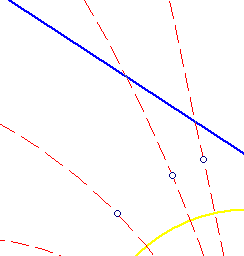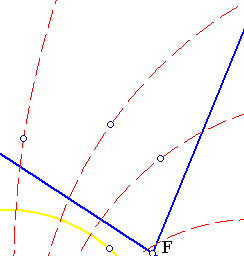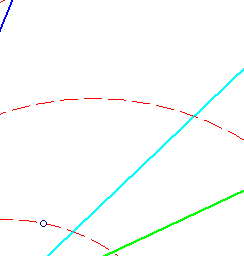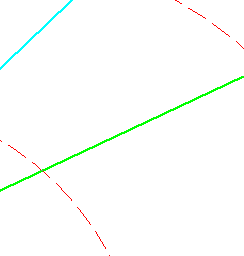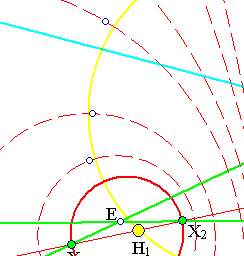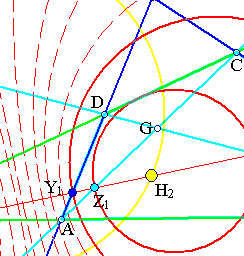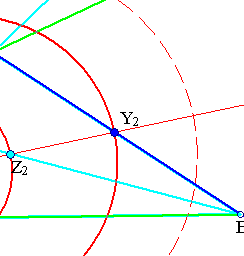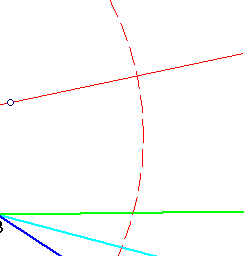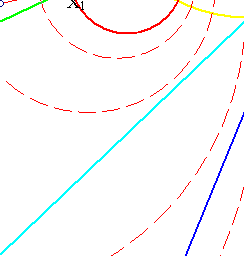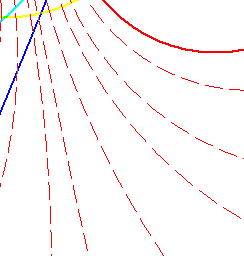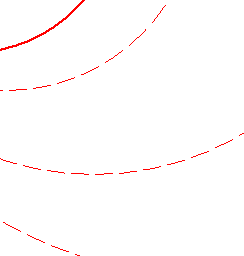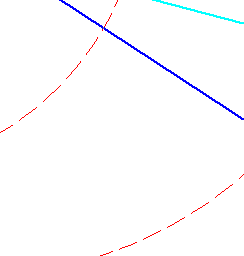In the reference, given above, it is proved that there is an involutive homography f on line (e) interchanging the elements of the pairs (X1,X2), (Y1,Y2) and (Z1,Z2). The rest follows from the discussion in Involution.html .
The fixed points H1, H2 of the involution are real in the case the circle bundle is of non-intersecting type. In that case they coincide with the limit points of the bundle. In the case the resulting bundle is of intersecting type H1, H2 are complex points. The file InvolutionBasic3.html contains a picture of that case.
The remarks made here can be useful for the determination of the fixed points of the involution (if any). This, for example, can be used to construct the conic passing through four points and tangent to a line, see FourPtsAndTangent.html .

### See Also

DesarguesInvolution.html
DesarguesInvolution2.html
DesarguesInvolution3.html
DesarguesInvolutionComplex.html
FourPtsAndTangent.html
Involution.html
InvolutionBasic2.html
InvolutionBasic3.html

 Produced with EucliDraw© http://users.math.uoc.gr/~pamfilos/